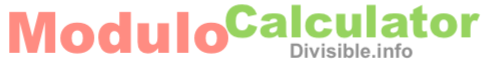What is 33 mod 13?Here we will explain what 33 mod 13 means and show how to calculate it. 33 mod 13 is short for 33 modulo 13 and it can also be called 33 modulus 13.

Modulo is the operation of finding the Remainder when you divide two numbers. Therefore, when you ask "What is 33 mod 13?" you are asking "What is the Remainder when you divide 33 by 13?".

We will show you two methods of finding 33 mod 13 (33 modulo 13). To differentiate our methods, we will call them the "Modulo Method" and the "Modulus Method".

Before we continue, we remind you of what the different parts of a division problem are called so you can follow along: Dividend / Divisor = Quotient and in this case 33 is the Dividend, 13 is the Divisor, and the answer is called the Quotient.

Furthermore, the Quotient x.y has two parts: x to the left of the decimal point is the Whole part, and y to the right of the decimal point is the Fractional part.

Modulo Method
To find 33 mod 13 using the Modulo Method, we first divide the Dividend (33) by the Divisor (13).

Second, we multiply the Whole part of the Quotient in the previous step by the Divisor (13).

Then finally, we subtract the answer in the second step from the Dividend (33) to get the answer. Here is the math to illustrate how to get 33 mod 13 using our Modulo Method:

33 / 13 = 2.538462
2 x 13 = 26
33 - 26 = 7

Thus, the answer to "What is 33 mod 13?" is 7.

Modulus Method
To find 33 mod 13 using the Modulus Method, we first find the highest multiple of the Divisor (13) that is equal to or less than the Dividend (33).

Then, we subtract the highest Divisor multiple from the Dividend to get the answer to 33 modulus 13 (33 mod 13):

Multiples of 13 are 0, 13, 26, 39, etc. and the highest multiple of 13 equal to or less than 33 is 26. Therefore, to get the answer:

33 - 26 = 7

Thus, once again, the answer to "What is 33 mod 13?" is 7.

Modulo Calculator
Do you have another "mod" problem you need solved? If so, please enter it here.

mod

What is 33 mod 14?
Go here to get the Modulo for the next problem in our math solution book.